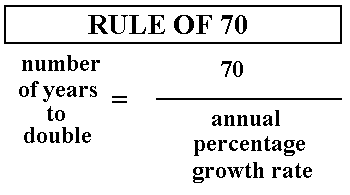# What is the Rule of 70 ?Ever wondered, how it is calculated that an economy will double in X years when it is growing at R% annually. Obviously we can simply use compound interest and calculate the number of periods it will take to double, however, there is a far simpler methodology to meet the same purpose. This method is referred as the Rule of 70. Let us see how this actually works:

If we are to calculate the period it will take for the amount to grow by 50%, we can calculate it by using ln 1.5/ln (1+r). If we generalise the format, it looks like ln (1+x)/ln(1+r), where x is percentage growth expected and r is the rate at which the investment is growing. If we are to calculate the number of periods (T) for an amount to double at a rate of interest r, the number of periods is given by T= ln 2/ ln (1+r). For simple interest, the value that we get is very close to 72/r , thereby signifying the rule of 72.For continuous compounding, the value is close to 69/r, also known as the rule of 69. In order to round it up, for common usage we normally take the rule of 70 to calculate the number of periods it takes for an amount to double. If we use the formula using natural logarithms, it gives a more accurate figure, however for quick estimation this rule is highly preferred and is used by investors. Furthermore, if we look at the mathematical form of the rule of 70 it implies, T= 70/r, where r is the interest or growth rate.  This rule is essentially helpful for economic growth predictions, which are normally characterised by less than 10% growth. For instance, the US economy growing at 2% will take 35 years to double itself, whereas the Indian economy at a growth rate of 7% will take 10 years to double itself. Here again, it must be kept in mind that this rule is derivation from compound interest calculation.

The Rule of 70 has another major use in calculating the period in which the money power gets reduced by half. In this case, instead of using the rate of interest, we use the inflation rate.  Say, for India the inflation rate is 10%, applying the Rule of 70, we get:

T= 70/10=7 years.

Hence in a period of 7 years if we have Rs. 100 today, it will be worth Rs. 50 based on this approach which is quite close to the value we get of 51.31 if we use a discount rate equal to the inflation rate. The problem with rule of 70 arises when r increases to higher value like 20% or higher, then the difference between approximate value calculated using this approach and the actual value increases to significant levels. In order to deal with such scenarios, there are several corrections like E-M rule and Pade approximant which introduces a correction factor to this approach to keep error within 5% level for higher values of rate.

Using E-M rule, the rule of 70 gets modified as: T= 70/r * 198/(200-r)

However, the rule of 70 is strictly meant to approximate values in a very simple way, so it is  only advised in scenarios where high level of accuracy is not required.

You will love reading: Indian Entrepreneur?.  Must read article How to join FMS?